### IFNA Function Examples – Excel, VBA, & Google Sheets

This tutorial demonstrates how to use the Excel IFNA Function in Excel to so something if a calculation results in an #N/A error.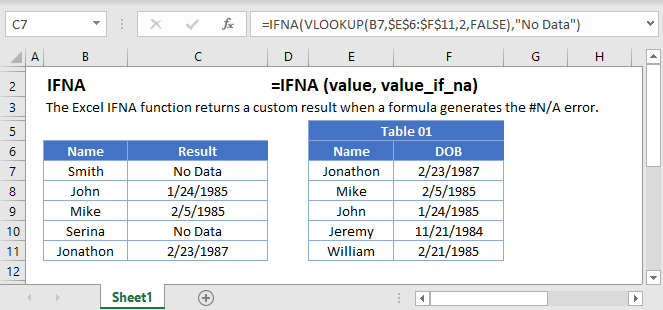## IFNA Function Overview

The IFNA Function tests if a calculation results in an #N/A Error. If not, it displays the calculation result. If so, it performs another calculation.

To use the IFNA Excel Worksheet Function, select a cell and type: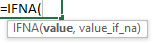(Notice how the formula inputs appear)

### IFNA Function Syntax and Inputs:

`=IFNA(value,value_if_na)`

value – A value, calculation, or cell reference.

num_digits – What to do if value results in an #N/A error.

## How to use the IFNA Function

The IFNA Function tests if a calculation results in a #N/A error, outputting a different value (or calculation) if an error is detected.

`=IFNA(VLOOKUP(A2,\$E\$2:\$F\$7,2,FALSE),"No Data")`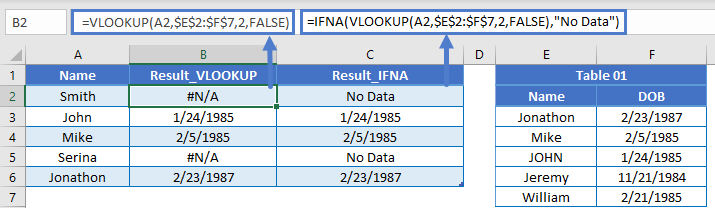### IFERROR Function

Alternatively, the IFERROR Function tests if any error occurs, including #N/A Errors.

```=IFERROR(A2,"ERROR_Found")
=IFNA(A2,"ERROR_Found")```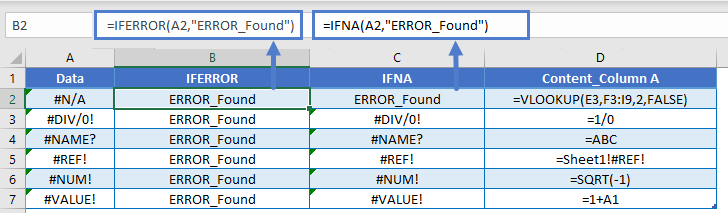### Why Use the IFNA Function?

You might be wondering, why should I just use the IFERROR Function? Well when working with lookup functions (like the VLOOKUP), if the lookup value isn’t found the function will return an #N/A.

`=IFERROR(VLOOKUP(F3,\$A\$3:\$D\$7,4,FALSE),"")`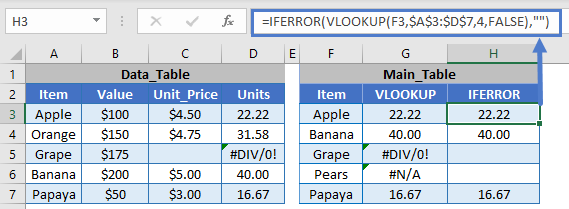You might only want to handle these valid #N/A errors by setting error values to zero or blank.

`=IFNA(VLOOKUP(F3,\$A\$3:\$D\$7,4,FALSE),"")`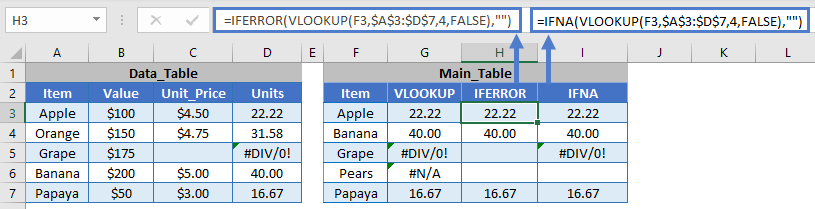But regular errors will still be shown, allowing you to identify if there is a calculation error with the formula.

The IFNA Function works exactly the same in Google Sheets as in Excel: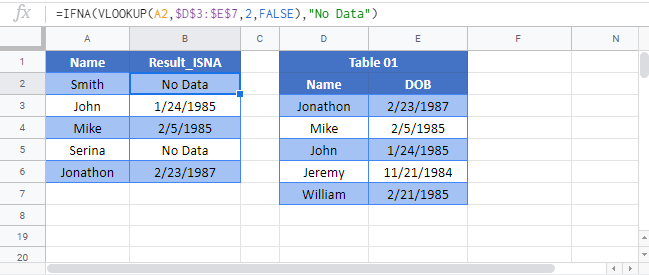## IFNA Examples in VBA

You can also use the IFNA function in VBA. Type:
`application.worksheetfunction.IFNA(value, value_if_na)`
For the function arguments (number, etc.), you can either enter them directly into the function, or define variables to use instead.

## Excel Practice Worksheet

Practice Excel functions and formulas with our 100% free practice worksheets!